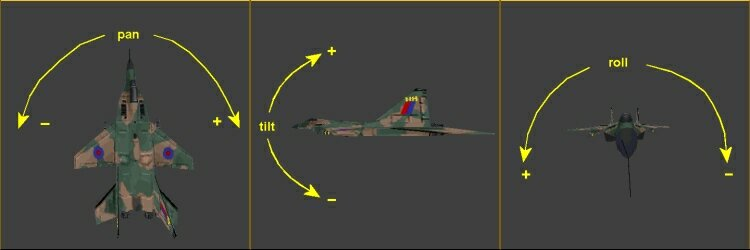## roll

The Euler angles that describe rotations about the Z, Y, and X axis (in degrees, 0..360). An entity can be rotated by changing these values.pan: 0 .. 360
tilt: -90 .. +90
roll: 0 .. 360

### Type:

var; entity.pan can also serve as ANGLE vector.

### Remarks:

• Angles are given in degrees (0 to 360) and counted counter-clockwise. For rotations in three dimensions the so-called Euler angles are used: pan is the horizontal angle (0..360) about the upright Z axis, tilt is the vertical angle (-90..+90) about the rotated Y axis, and roll is the angle (0..360) about the rotated and tilted X axis (see image). The zero direction (pan = 0, tilt = 0, roll = 0) runs along the X-axis (east). pan = 90 is north, pan = 180 is west, and pan = 270 is south.
• By changing an Euler angle, the entity rotates about the axes of the world coordinate system. This is not the same as rotating the entity about it's own axes that may be arbitrarily tilted when the entity was rotated byfore. For rotating an entity about it's own axis, which is often required for flight or spaceship simulators, use the ang_add or ang_rotate functions.
• For orienting the entity in a certain direction or towards a certain point in space, use the vec_to_angle function.
• Angles have a special meaning with sprites. If all angles are at zero, the sprite will stand upright and horizontally face the camera. If its pan or tilt angle is nonzero, the sprite is oriented in world space according to its angles. If pan and tilt both are 0, but the roll angle is nonzero, the sprite is always perpendicular to the camera. This is useful for spherical objects, like fireballs or explosions. For an angle to be nonzero it's sufficient to set it at a small amount, like 0.01.
• Exceeding the angle ranges given above is possible. But be aware of ambiguities in entity orientation, and of 'gimbal lock' problems. If you don't know what angle ambiguities and gimbal lock problems are, better don't deal with them and don't exceed the angle limits.
• Don't change entity angles more than once per frame. This could otherwise cause problems with intermediate rotations because Euler angles are internally converted to a rotation matrix resp. a quaternion.
• Other coordinate systems require different angles for the same rotation. For instance, DirectX uses a left-handed coordinate system with the Y-axis standing upright, and the zero direction running along the Z axis (north). For converting an Euler view angle to a DirectX view rotation matrix, first rotate by pan minus 90 degrees about the DirectX Y axis, then by tilt about the DirectX X axis, then by roll about the DirectX Z axis. For converting an Euler object rotation to a DirectX object rotation matrix, rotate by -roll about the X axis, then by tilt about the Z axis, then by -pan about the Y axis (see also ang_to_matrix).

### Example:

```action rotator(){
while(1)
{
my.pan += time_step;
wait(1);
}
}
```